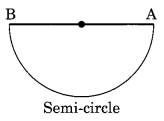On this page, you will find Basic Geometrical Ideas Class 6 Notes Maths Chapter 4 Pdf free download. CBSE NCERT Class 6 Maths Notes Chapter 4 Basic Geometrical Ideas will seemingly help them to revise the important concepts in less time.

## CBSE Class 6 Maths Chapter 4 Notes Basic Geometrical Ideas

### Basic Geometrical Ideas Class 6 Notes Conceptual Facts

1. Basic Geometrical Elements are points, lines and planes.

2. Point determines a location. It does not have length, breadth and thickness. It is usually denoted by a capital letter.3. Line segment is the shortest distance between two points. Line segment joining points A and B is denoted by AB. AB and BA denote the same line segment.
$$\overline{\mathrm{AB}} \cdot \overline{\mathrm{AB}} \text { and } \overline{\mathrm{BA}}$$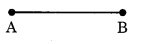4. When a line segment is extended on both sides infinitely we get a line and it is denoted by $$\overline{\mathrm{AB}}$$.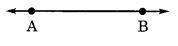5. A ray is a portion of line starting at a point and extending in one direction endlessly. It is denoted by$$\overline{\mathrm{AB}}$$.6. Two distinct lines which meet at a point are called intersecting lines.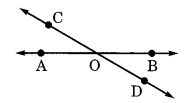7. Two distinct lines which do not meet at any point, are called parallel lines and denoted by
|| i.e., $$\overline{\mathrm{AB}} \| \overline{\mathrm{CD}}$$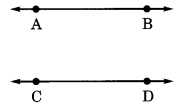8. Any drawing (straight or non-straight) drawn without lifting the pencil is called a curve.9. Simple curve does not intersects itself.

10. If the end points of a simple curve are joined, then it is called as closed curve.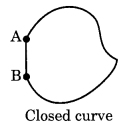11. If the end points of a curve are not joined, then it is called as open curve.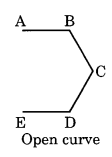12. Polygon is a simple closed curve made up of line segments.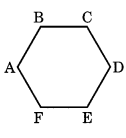13. An angle is made up of two rays starting from a common end point. It is read as ∠BOA or ∠AOB.14. Angle has three regions: On the angle, the interior of the angle and the exterior of the angle.

15. Triangle is a polygon of three sides denoted by ΔABC.16. Quadrilateral is polygon of four sides.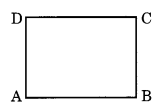17. Circle is a path of a point moving at the same distance from a fixed point known as centre and the distance between the fixed point and the moving point is called radius.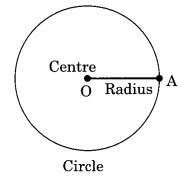18. Chord of a circle is a line segment obtained by joining any two points on the circle. It divides the circle into two segments, minor segment and major segment.19. Diameter is the longest chord of a circle which passes through the centre and divides the circle into two semi-circles.